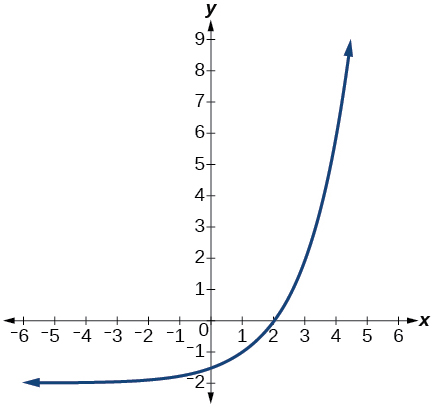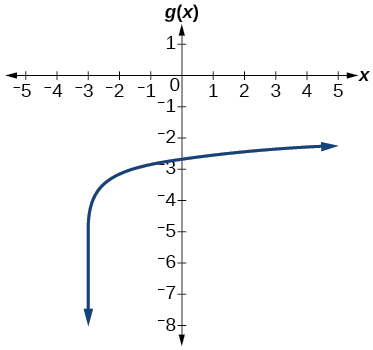# 6.8 Fitting exponential models to data  (Page 10/12)

 Page 10 / 12

The graph below shows transformations of the graph of $\text{\hspace{0.17em}}f\left(x\right)={2}^{x}.\text{\hspace{0.17em}}$ What is the equation for the transformation?## Logarithmic Functions

Rewrite $\text{\hspace{0.17em}}{\mathrm{log}}_{17}\left(4913\right)=x\text{\hspace{0.17em}}$ as an equivalent exponential equation.

${17}^{x}=4913$

Rewrite $\text{\hspace{0.17em}}\mathrm{ln}\left(s\right)=t\text{\hspace{0.17em}}$ as an equivalent exponential equation.

Rewrite $\text{\hspace{0.17em}}{a}^{-\text{\hspace{0.17em}}\frac{2}{5}}=b\text{\hspace{0.17em}}$ as an equivalent logarithmic equation.

${\mathrm{log}}_{a}b=-\frac{2}{5}$

Rewrite $\text{\hspace{0.17em}}{e}^{-3.5}=h\text{\hspace{0.17em}}$ as an equivalent logarithmic equation.

Solve for $\text{\hspace{0.17em}}x\text{\hspace{0.17em}}\text{\hspace{0.17em}}{\mathrm{log}}_{64}\left(x\right)=\frac{1}{3}\text{\hspace{0.17em}}$ to exponential form.

$x={64}^{\frac{1}{3}}=4$

Evaluate $\text{\hspace{0.17em}}{\mathrm{log}}_{5}\left(\frac{1}{125}\right)\text{\hspace{0.17em}}$ without using a calculator.

Evaluate $\text{\hspace{0.17em}}\mathrm{log}\left(\text{0}\text{.000001}\right)\text{\hspace{0.17em}}$ without using a calculator.

$\mathrm{log}\left(\text{0}\text{.000001}\right)=-6$

Evaluate $\text{\hspace{0.17em}}\mathrm{log}\left(4.005\right)\text{\hspace{0.17em}}$ using a calculator. Round to the nearest thousandth.

Evaluate $\text{\hspace{0.17em}}\mathrm{ln}\left({e}^{-0.8648}\right)\text{\hspace{0.17em}}$ without using a calculator.

$\mathrm{ln}\left({e}^{-0.8648}\right)=-0.8648$

Evaluate $\text{\hspace{0.17em}}\mathrm{ln}\left(\sqrt{18}\right)\text{\hspace{0.17em}}$ using a calculator. Round to the nearest thousandth.

## Graphs of Logarithmic Functions

Graph the function $\text{\hspace{0.17em}}g\left(x\right)=\mathrm{log}\left(7x+21\right)-4.$Graph the function $\text{\hspace{0.17em}}h\left(x\right)=2\mathrm{ln}\left(9-3x\right)+1.$

State the domain, vertical asymptote, and end behavior of the function $\text{\hspace{0.17em}}g\left(x\right)=\mathrm{ln}\left(4x+20\right)-17.$

Domain: $\text{\hspace{0.17em}}x>-5;\text{\hspace{0.17em}}$ Vertical asymptote: $\text{\hspace{0.17em}}x=-5;\text{\hspace{0.17em}}$ End behavior: as $\text{\hspace{0.17em}}x\to -{5}^{+},f\left(x\right)\to -\infty \text{\hspace{0.17em}}$ and as $\text{\hspace{0.17em}}x\to \infty ,f\left(x\right)\to \infty .$

## Logarithmic Properties

Rewrite $\text{\hspace{0.17em}}\mathrm{ln}\left(7r\cdot 11st\right)\text{\hspace{0.17em}}$ in expanded form.

Rewrite $\text{\hspace{0.17em}}{\mathrm{log}}_{8}\left(x\right)+{\mathrm{log}}_{8}\left(5\right)+{\mathrm{log}}_{8}\left(y\right)+{\mathrm{log}}_{8}\left(13\right)\text{\hspace{0.17em}}$ in compact form.

${\text{log}}_{8}\left(65xy\right)$

Rewrite $\text{\hspace{0.17em}}{\mathrm{log}}_{m}\left(\frac{67}{83}\right)\text{\hspace{0.17em}}$ in expanded form.

Rewrite $\text{\hspace{0.17em}}\mathrm{ln}\left(z\right)-\mathrm{ln}\left(x\right)-\mathrm{ln}\left(y\right)\text{\hspace{0.17em}}$ in compact form.

$\mathrm{ln}\left(\frac{z}{xy}\right)$

Rewrite $\text{\hspace{0.17em}}\mathrm{ln}\left(\frac{1}{{x}^{5}}\right)\text{\hspace{0.17em}}$ as a product.

Rewrite $\text{\hspace{0.17em}}-{\mathrm{log}}_{y}\left(\frac{1}{12}\right)\text{\hspace{0.17em}}$ as a single logarithm.

${\text{log}}_{y}\left(12\right)$

Use properties of logarithms to expand $\text{\hspace{0.17em}}\mathrm{log}\left(\frac{{r}^{2}{s}^{11}}{{t}^{14}}\right).$

Use properties of logarithms to expand $\text{\hspace{0.17em}}\mathrm{ln}\left(2b\sqrt{\frac{b+1}{b-1}}\right).$

$\mathrm{ln}\left(2\right)+\mathrm{ln}\left(b\right)+\frac{\mathrm{ln}\left(b+1\right)-\mathrm{ln}\left(b-1\right)}{2}$

Condense the expression $\text{\hspace{0.17em}}5\mathrm{ln}\left(b\right)+\mathrm{ln}\left(c\right)+\frac{\mathrm{ln}\left(4-a\right)}{2}\text{\hspace{0.17em}}$ to a single logarithm.

Condense the expression $\text{\hspace{0.17em}}3{\mathrm{log}}_{7}v+6{\mathrm{log}}_{7}w-\frac{{\mathrm{log}}_{7}u}{3}\text{\hspace{0.17em}}$ to a single logarithm.

${\mathrm{log}}_{7}\left(\frac{{v}^{3}{w}^{6}}{\sqrt{u}}\right)$

Rewrite $\text{\hspace{0.17em}}{\mathrm{log}}_{3}\left(12.75\right)\text{\hspace{0.17em}}$ to base $\text{\hspace{0.17em}}e.$

Rewrite $\text{\hspace{0.17em}}{5}^{12x-17}=125\text{\hspace{0.17em}}$ as a logarithm. Then apply the change of base formula to solve for $\text{\hspace{0.17em}}x\text{\hspace{0.17em}}$ using the common log. Round to the nearest thousandth.

$x=\frac{\frac{\mathrm{log}\left(125\right)}{\mathrm{log}\left(5\right)}+17}{12}=\frac{5}{3}$

## Exponential and Logarithmic Equations

Solve $\text{\hspace{0.17em}}{216}^{3x}\cdot {216}^{x}={36}^{3x+2}\text{\hspace{0.17em}}$ by rewriting each side with a common base.

Solve $\text{\hspace{0.17em}}\frac{125}{{\left(\frac{1}{625}\right)}^{-x-3}}={5}^{3}\text{\hspace{0.17em}}$ by rewriting each side with a common base.

$x=-3$

Use logarithms to find the exact solution for $\text{\hspace{0.17em}}7\cdot {17}^{-9x}-7=49.\text{\hspace{0.17em}}$ If there is no solution, write no solution .

Use logarithms to find the exact solution for $\text{\hspace{0.17em}}3{e}^{6n-2}+1=-60.\text{\hspace{0.17em}}$ If there is no solution, write no solution .

no solution

Find the exact solution for $\text{\hspace{0.17em}}5{e}^{3x}-4=6\text{\hspace{0.17em}}$ . If there is no solution, write no solution .

Find the exact solution for $\text{\hspace{0.17em}}2{e}^{5x-2}-9=-56.\text{\hspace{0.17em}}$ If there is no solution, write no solution .

no solution

Find the exact solution for $\text{\hspace{0.17em}}{5}^{2x-3}={7}^{x+1}.\text{\hspace{0.17em}}$ If there is no solution, write no solution .

Find the exact solution for $\text{\hspace{0.17em}}{e}^{2x}-{e}^{x}-110=0.\text{\hspace{0.17em}}$ If there is no solution, write no solution .

$x=\mathrm{ln}\left(11\right)$

Use the definition of a logarithm to solve. $\text{\hspace{0.17em}}-5{\mathrm{log}}_{7}\left(10n\right)=5.$

47. Use the definition of a logarithm to find the exact solution for $\text{\hspace{0.17em}}9+6\mathrm{ln}\left(a+3\right)=33.$

$a={e}^{4}-3$

Use the one-to-one property of logarithms to find an exact solution for $\text{\hspace{0.17em}}{\mathrm{log}}_{8}\left(7\right)+{\mathrm{log}}_{8}\left(-4x\right)={\mathrm{log}}_{8}\left(5\right).\text{\hspace{0.17em}}$ If there is no solution, write no solution .

Use the one-to-one property of logarithms to find an exact solution for $\text{\hspace{0.17em}}\mathrm{ln}\left(5\right)+\mathrm{ln}\left(5{x}^{2}-5\right)=\mathrm{ln}\left(56\right).\text{\hspace{0.17em}}$ If there is no solution, write no solution .

$x=±\frac{9}{5}$

The formula for measuring sound intensity in decibels $\text{\hspace{0.17em}}D\text{\hspace{0.17em}}$ is defined by the equation $\text{\hspace{0.17em}}D=10\mathrm{log}\left(\frac{I}{{I}_{0}}\right),$ where $\text{\hspace{0.17em}}I\text{\hspace{0.17em}}$ is the intensity of the sound in watts per square meter and $\text{\hspace{0.17em}}{I}_{0}={10}^{-12}\text{\hspace{0.17em}}$ is the lowest level of sound that the average person can hear. How many decibels are emitted from a large orchestra with a sound intensity of $\text{\hspace{0.17em}}6.3\cdot {10}^{-3}\text{\hspace{0.17em}}$ watts per square meter?

#### Questions & Answers

A laser rangefinder is locked on a comet approaching Earth. The distance g(x), in kilometers, of the comet after x days, for x in the interval 0 to 30 days, is given by g(x)=250,000csc(π30x). Graph g(x) on the interval [0, 35]. Evaluate g(5)  and interpret the information. What is the minimum distance between the comet and Earth? When does this occur? To which constant in the equation does this correspond? Find and discuss the meaning of any vertical asymptotes.
Kaitlyn Reply
The sequence is {1,-1,1-1.....} has
amit Reply
circular region of radious
Kainat Reply
how can we solve this problem
Joel Reply
Sin(A+B) = sinBcosA+cosBsinA
Eseka Reply
Prove it
Eseka
Please prove it
Eseka
hi
Joel
June needs 45 gallons of punch. 2 different coolers. Bigger cooler is 5 times as large as smaller cooler. How many gallons in each cooler?
Arleathia Reply
7.5 and 37.5
Nando
find the sum of 28th term of the AP 3+10+17+---------
Prince Reply
I think you should say "28 terms" instead of "28th term"
Vedant
the 28th term is 175
Nando
192
Kenneth
if sequence sn is a such that sn>0 for all n and lim sn=0than prove that lim (s1 s2............ sn) ke hole power n =n
SANDESH Reply
write down the polynomial function with root 1/3,2,-3 with solution
Gift Reply
if A and B are subspaces of V prove that (A+B)/B=A/(A-B)
Pream Reply
write down the value of each of the following in surd form a)cos(-65°) b)sin(-180°)c)tan(225°)d)tan(135°)
Oroke Reply
Prove that (sinA/1-cosA - 1-cosA/sinA) (cosA/1-sinA - 1-sinA/cosA) = 4
kiruba Reply
what is the answer to dividing negative index
Morosi Reply
In a triangle ABC prove that. (b+c)cosA+(c+a)cosB+(a+b)cisC=a+b+c.
Shivam Reply
give me the waec 2019 questions
Aaron Reply

### Read also:

#### Get the best Algebra and trigonometry course in your pocket!

Source:  OpenStax, Algebra and trigonometry. OpenStax CNX. Nov 14, 2016 Download for free at https://legacy.cnx.org/content/col11758/1.6
Google Play and the Google Play logo are trademarks of Google Inc.

Notification Switch

Would you like to follow the 'Algebra and trigonometry' conversation and receive update notifications?ByByBy Lakeima RobertsBy Anonymous UserBy RhodesBy Abishek DevarajBy Edward Biton